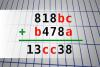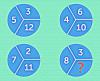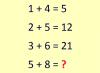BRAIN TEASERSBrain Teasers User Profile

# bipinraj kunwar

rank
355
points
7
See full ranking list
short ranking list
 353 sneška se smeška 7 354 Alexis StLaurent 7 355 bipinraj kunwar 7 356 Alexandra Santos 7 357 Anh Phạm 7
 Find number abc If 818bc + b478a = 13cc38 find number abc. Multiple solutions may exist.MATH PUZZLE: What is the mis... MATH PUZZLE: What is the missing number?Calculate 5+8 If 1+4=5, 2+5=12 and 3+6=21 then 5+8=?